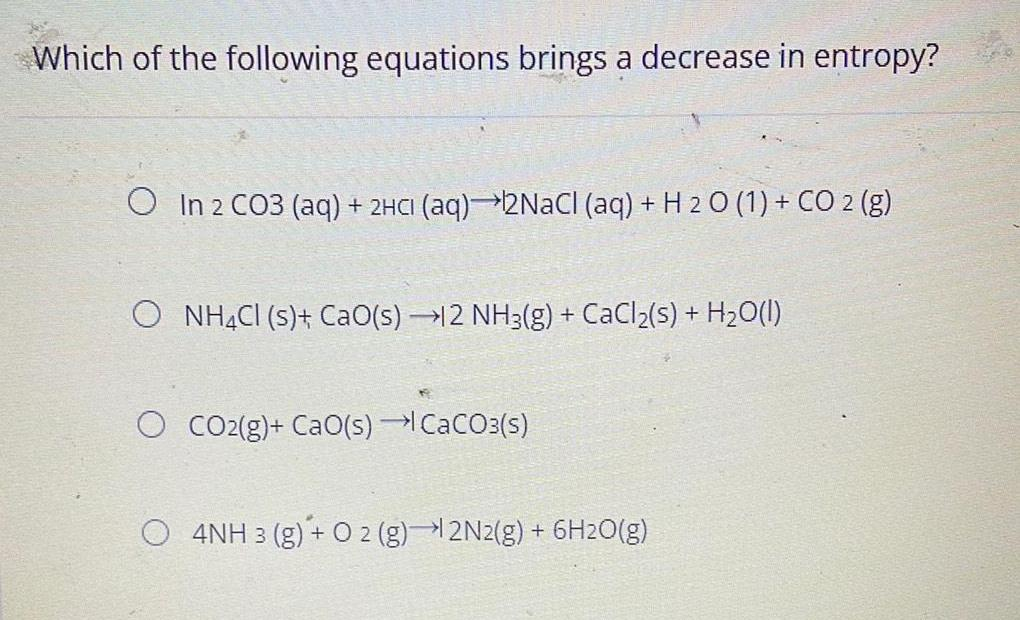Question:

# Which of the following equations brings a decrease in

Last updated: 8/8/2022Which of the following equations brings a decrease in entropy? In 2 CO3 (aq) + 2HCI (aq)→2NaCl (aq) + H2O(l) + C2 (g) NH4Cl (s)+ CaO(s) →12 NH3(g) + CaCl₂(s) + H₂O(l) CO2(g) + CaO(s) → CaCO3(s) 4NH 3 (g) + O2 (g)→ 2N2(g) + 6H2O(g)